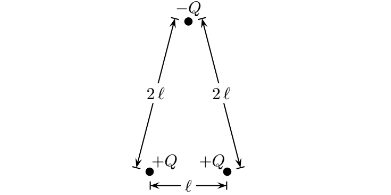Problem: A system of charges are shown in the figure. What is the electric potential energy of the following system of charges? 1. U = kQ2 / ℓ 2. U = 0 3. U = kQ2 / 3ℓ 4. U = kQ2 / ℓ2 5. U = - kQ2 / 2ℓ 6. U = - kQ2 / ℓ 7. U = - kQ2 / 3ℓ 8. U = kQ2 9. None of these. 10. U = kQ2 / 2ℓ

99% (81 ratings)
Problem Details

A system of charges are shown in the figure. What is the electric potential energy of the following system of charges?

1. U = kQ2 / ℓ

2. U = 0

3. UkQ/ 3ℓ

4. UkQ/ ℓ2

5. U = - kQ/ 2ℓ

6. U = - kQ/ ℓ

7. U = - kQ/ 3ℓ

8. UkQ2

9. None of these.

10. U = kQ/ 2ℓ